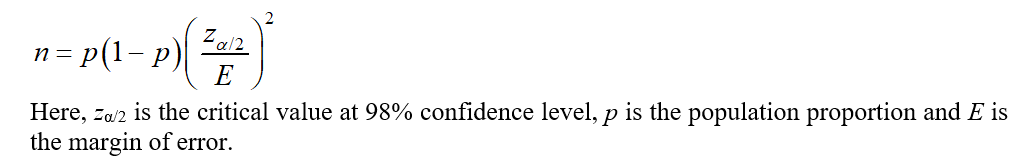# You want to obtain a sample to estimate a population proportion. Based on previous evidence, you believe the population proportion is approximately p∗=65%p∗=65%. You would like to be 98% confident that your esimate is within 0.1% of the true population proportion. How large of a sample size is required?n =

Question
10 views

You want to obtain a sample to estimate a population proportion. Based on previous evidence, you believe the population proportion is approximately
p

=
65
%
p∗=65%
. You would like to be 98% confident that your esimate is within 0.1% of the true population proportion. How large of a sample size is required?

n =

check_circle

Step 1

The formula for the sample size is as follows:...

### Want to see the full answer?

See Solution

#### Want to see this answer and more?

Solutions are written by subject experts who are available 24/7. Questions are typically answered within 1 hour.*

See Solution
*Response times may vary by subject and question.
Tagged in